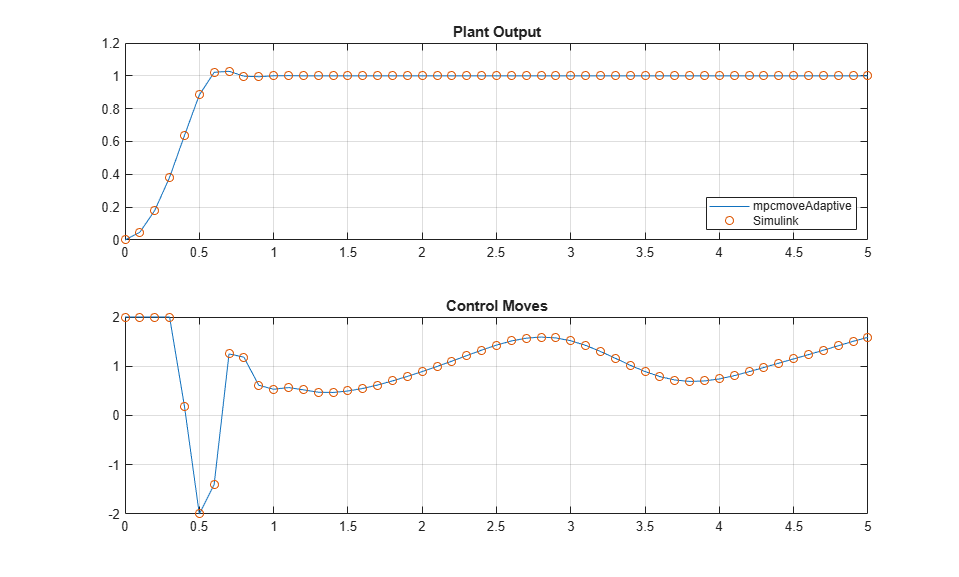Documentation

## Time-Varying MPC Control of a Time-Varying Plant

This example shows how the Model Predictive Control Toolbox™ can use time-varying prediction models to achieve better performance when controlling a time-varying plant.

The following MPC controllers are compared:

1. Linear MPC controller based on a time-invariant average model

2. Linear MPC controller based on a time-invariant model, which is updated at each time step.

3. Linear MPC controller based on a time-varying prediction model.

### Time-Varying Linear Plant

In this example, the plant is a single-input-single-output 3rd order time-varying linear system with poles, zeros and gain that vary periodically with time.

``

The plant poles move between being stable and unstable at run time, which leads to a challenging control problem.

Generate an array of plant models at `t` = `0`, `0.1`, `0.2`, ..., 10 seconds.

```Models = tf; ct = 1; for t = 0:0.1:10 Models(:,:,ct) = tf([5 5+2*cos(2.5*t)],[1 3 2 6+sin(5*t)]); ct = ct + 1; end ```

Convert the models to state-space format and discretize them with a sample time of 0.1 second.

```Ts = 0.1; Models = ss(c2d(Models,Ts)); ```

### MPC Controller Design

The control objective is to track a step change in the reference signal. First, design an MPC controller for the average plant model. The controller sample time is 0.1 second.

```sys = ss(c2d(tf([5 5],[1 3 2 6]),Ts)); % prediction model p = 3; % prediction horizon m = 3; % control horizon mpcobj = mpc(sys,Ts,p,m); ```
```-->The "Weights.ManipulatedVariables" property of "mpc" object is empty. Assuming default 0.00000. -->The "Weights.ManipulatedVariablesRate" property of "mpc" object is empty. Assuming default 0.10000. -->The "Weights.OutputVariables" property of "mpc" object is empty. Assuming default 1.00000. ```

Set hard constraints on the manipulated variable and specify tuning weights.

```mpcobj.MV = struct('Min',-2,'Max',2); mpcobj.Weights = struct('MV',0,'MVRate',0.01,'Output',1); ```

Set the initial plant states to zero.

```x0 = zeros(size(sys.B)); ```

### Closed-Loop Simulation with Implicit MPC

Run a closed-loop simulation to examine whether the designed implicit MPC controller can achieve the control objective without updating the plant model used in prediction.

Set the simulation duration to 5 seconds.

```Tstop = 5; ```

Use the `mpcmove` command in a loop to simulate the closed-loop response.

```yyMPC = []; uuMPC = []; x = x0; xmpc = mpcstate(mpcobj); fprintf('Simulating MPC controller based on average LTI model.\n'); for ct = 1:(Tstop/Ts+1) % Get the real plant. real_plant = Models(:,:,ct); % Update and store the plant output. y = real_plant.C*x; yyMPC = [yyMPC,y]; % Compute and store the MPC optimal move. u = mpcmove(mpcobj,xmpc,y,1); uuMPC = [uuMPC,u]; % Update the plant state. x = real_plant.A*x + real_plant.B*u; end ```
```-->Assuming output disturbance added to measured output channel #1 is integrated white noise. -->The "Model.Noise" property of the "mpc" object is empty. Assuming white noise on each measured output channel. Simulating MPC controller based on average LTI model. ```

### Closed-Loop Simulation with Adaptive MPC

Run a second simulation to examine whether an adaptive MPC controller can achieve the control objective.

Use the `mpcmoveAdaptive` command in a loop to simulate the closed-loop response. Update the plant model for each control interval, and use the updated model to compute the optimal control moves. The `mpcmoveAdaptive` command uses the same prediction model across the prediction horizon.

```yyAMPC = []; uuAMPC = []; x = x0; xmpc = mpcstate(mpcobj); nominal = mpcobj.Model.Nominal; fprintf('Simulating MPC controller based on LTI model, updated at each time step t.\n'); for ct = 1:(Tstop/Ts+1) % Get the real plant. real_plant = Models(:,:,ct); % Update and store the plant output. y = real_plant.C*x; yyAMPC = [yyAMPC, y]; % Compute and store the MPC optimal move. u = mpcmoveAdaptive(mpcobj,xmpc,real_plant,nominal,y,1); uuAMPC = [uuAMPC,u]; % Update the plant state. x = real_plant.A*x + real_plant.B*u; end ```
```Simulating MPC controller based on LTI model, updated at each time step t. ```

### Closed-Loop Simulation with Time-Varying MPC

Run a third simulation to examine whether a time-varying MPC controller can achieve the control objective.

The controller updates the prediction model at each control interval and also uses time-varying models across the prediction horizon, which gives MPC controller the best knowledge of plant behavior in the future.

Use the `mpcmoveAdaptive` command in a loop to simulate the closed-loop response. Specify an array of plant models rather than a single model. The controller uses each model in the array at a different prediction horizon step.

```yyLTVMPC = []; uuLTVMPC = []; x = x0; xmpc = mpcstate(mpcobj); Nominals = repmat(nominal,3,1); % Nominal conditions are constant over the prediction horizon. fprintf('Simulating MPC controller based on time-varying model, updated at each time step t.\n'); for ct = 1:(Tstop/Ts+1) % Get the real plant. real_plant = Models(:,:,ct); % Update and store the plant output. y = real_plant.C*x; yyLTVMPC = [yyLTVMPC, y]; % Compute and store the MPC optimal move. u = mpcmoveAdaptive(mpcobj,xmpc,Models(:,:,ct:ct+p),Nominals,y,1); uuLTVMPC = [uuLTVMPC,u]; % Update the plant state. x = real_plant.A*x + real_plant.B*u; end ```
```Simulating MPC controller based on time-varying model, updated at each time step t. ```

### Performance Comparison of MPC Controllers

Compare the closed-loop responses.

```t = 0:Ts:Tstop; figure subplot(2,1,1); plot(t,yyMPC,'-.',t,yyAMPC,'--',t,yyLTVMPC); grid legend('Implicit MPC','Adaptive MPC','Time-Varying MPC','Location','SouthEast') title('Plant Output'); subplot(2,1,2) plot(t,uuMPC,'-.',t,uuAMPC,'--',t,uuLTVMPC) grid title('Control Moves'); ```Only the time-varying MPC controller is able to bring the plant output close enough to the desired setpoint.

### Closed-Loop Simulation of Time-Varying MPC in Simulink

To simulate time-varying MPC control in Simulink, pass the time-varying plant models to `model` inport of the Adaptive MPC Controller block.

```xmpc = mpcstate(mpcobj); mdl = 'mpc_timevarying'; open_system(mdl); ```Run the simulation.

```sim(mdl,Tstop); fprintf('Simulating MPC controller based on LTV model in Simulink.\n'); ```
```Simulating MPC controller based on LTV model in Simulink. ```

Plot the MATLAB and Simulink time-varying simulation results.

```figure subplot(2,1,1) plot(t,yyLTVMPC,t,ysim,'o'); grid legend('mpcmoveAdaptive','Simulink','Location','SouthEast') title('Plant Output'); subplot(2,1,2) plot(t,uuLTVMPC,t,usim,'o') grid title('Control Moves'); ```The closed-loop responses in MATLAB and Simulink are identical.

```bdclose(mdl); ```